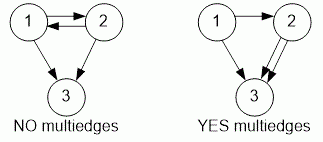Problems

# Multiedges

Time limit 1 second
Memory limit 128 MiB

A directed graph is given with a list of edges. Check whether it contains multiedges.

## Input data

The first line contains number of vertices in a graph n (1n100) and number of edges m (1m10000). Each of the next m lines contains pair of integers - the edges of the graph.

## Output data

Print YES if graph contains multiedges and NO otherwise.## Examples

Input example #1
3 4
1 2
2 3
1 3
2 1

Output example #1
NO

Input example #2
3 4
1 2
2 3
1 3
2 3

Output example #2
YES